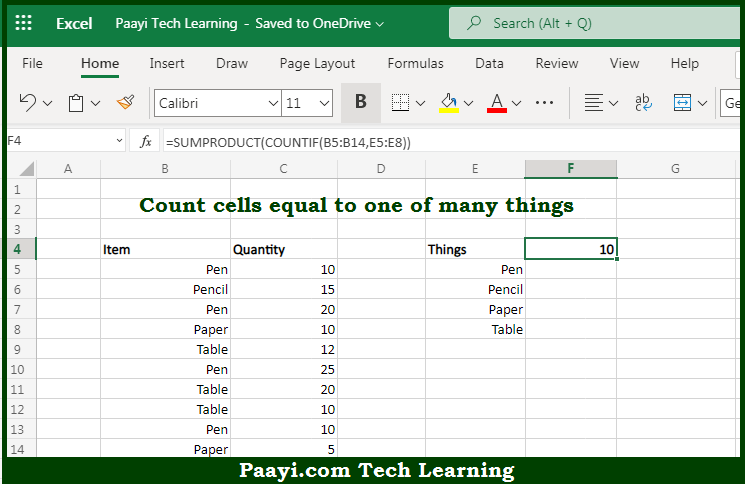# Learn How to Count Cells Equal to One of Many Things In Microsoft Excel

Written by | 0 Comments | 555 Views

In this article, you will learn how to use the Microsoft Excel COUNT formula and its purpose in Microsoft Excel. You will also get to know how to count cells equal to one of many things and see the generic formula.

Count Cells Equal to One of Many Things Using Microsoft Excel Count Formula

The main purpose of the Microsoft Excel COUNT formula is to count the number of cells equal to one of many values. Here we will learn how to count cells equal to one of many things.  That implies, with the help of the COUNT formula you can able to count the number of cells equal to one of many values. The COUNTIF function can be used inside of the SUMPRODUCT function. In the generic form of the formula below, rng represents a range of cells, and things represent the values to count. So, with the help of the COUNT formula, you can able to count the number of cells equal to one of many values.

General Syntax of the COUNT Cells Equal to Either x or y

=SUMPRODUCT(COUNTIF(rng,things))

The Explanation for the Count Cells Equal to Either x or ySo we know that Microsoft Excel COUNT formula you can able to count the number of cells equal to one of many values. Here we will learn how to count cells equal to one of many things. The COUNTIF function counts the number of cells in a range that meets the criteria. Whenever you provide COUNTIF with a range of cells as the criteria, it will return an array of numbers as the result. And, each number represents the count of one thing in the criteria range.

It should be noted that COUNTIF is not case-sensitive. So, with the help of the COUNT formula, you can able to count the number of cells equal to one of many values.